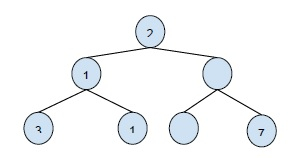# Print Binary Tree levels in sorted order in C++

In this problem, we are given a binary tree and we have to print all nodes at a level in sorted order of their values.

Let’s take an example to understand the concept better,

InputOutput

20
6 15
2 17 32 78

To solve this problem, we need to print a sorted order of each level of the tree. For this, we need to create a queue and two priority queues. The NULL separator is used to separate two levels.

## Example

Program to illustrate the logic −

Live Demo

#include <iostream>
#include <queue>
#include <vector>
using namespace std;
struct Node {
int data;
struct Node *left, *right;
};
void printLevelElements(Node* root){
if (root == NULL)
return;
queue<Node*> q;
priority_queue<int, vector<int>, greater<int> > current_level;
priority_queue<int, vector<int>, greater<int> > next_level;
q.push(root);
q.push(NULL);
current_level.push(root->data);
while (q.empty() == false) {
int data = current_level.top();
Node* node = q.front();
if (node == NULL) {
q.pop();
if (q.empty())
break;
q.push(NULL);
cout << "\n";
current_level.swap(next_level);
continue;
}
cout << data << " ";
q.pop();
current_level.pop();
if (node->left != NULL) {
q.push(node->left);
next_level.push(node->left->data);
}
if (node->right != NULL) {
q.push(node->right);
next_level.push(node->right->data);
}
}
}
Node* insertNode(int data){
Node* temp = new Node;
temp->data = data;
temp->left = temp->right = NULL;
return temp;
}
int main(){
Node* root = insertNode(12);
root->left = insertNode(98);
root->right = insertNode(34);
root->left->left = insertNode(76);
root->left->right = insertNode(5);
root->right->left = insertNode(12);
root->right->right = insertNode(45);
cout << "Elements at each Level of binary tree are \n";
printLevelElements(root);
return 0;
}

## Output

Elements at each Level of binary tree are
12
34 98
5 12 45 76